# Instrumentation Concepts Groundbased Optical Telescopes Keith Taylor IAGUSP

• Slides: 38
Download presentation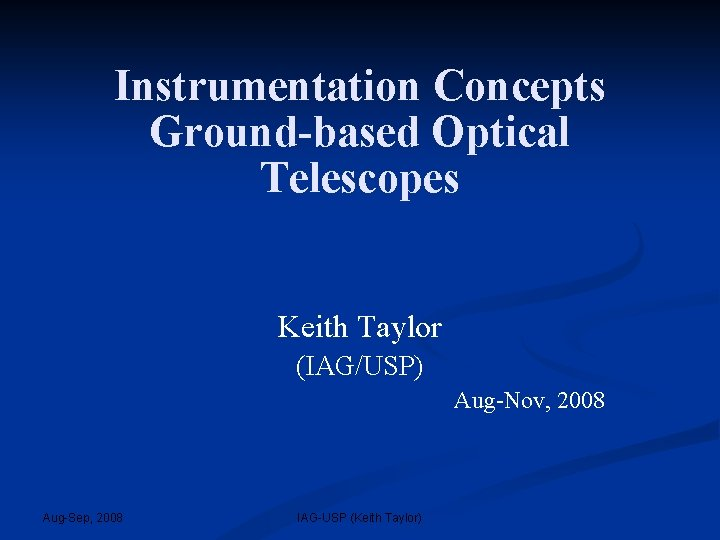Instrumentation Concepts Ground-based Optical Telescopes Keith Taylor (IAG/USP) Aug-Nov, 2008 Aug-Sep, 2008 IAG-USP (Keith Taylor)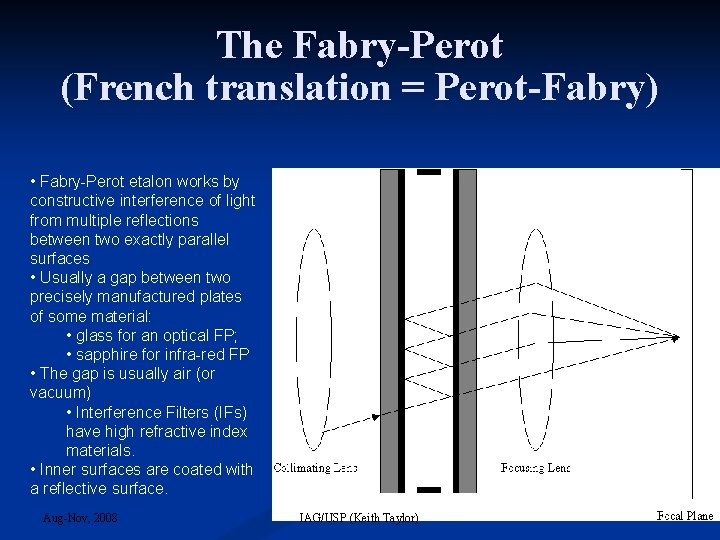The Fabry-Perot (French translation = Perot-Fabry) • Fabry-Perot etalon works by constructive interference of light from multiple reflections between two exactly parallel surfaces • Usually a gap between two precisely manufactured plates of some material: • glass for an optical FP; • sapphire for infra-red FP • The gap is usually air (or vacuum) • Interference Filters (IFs) have high refractive index materials. • Inner surfaces are coated with a reflective surface. Aug-Nov, 2008 IAG/USP (Keith Taylor)Fabry-Perot Light enters etalon and is subjected to multiple reflections Transmission spectrum has numerous narrow peaks at wavelengths where path difference results in constructive interference need ‘blocking filters’ to use as narrow band filter Width and depth of peaks depends on reflectivity of etalon surfaces: finesse Aug-Nov, 2008 IAG/USP (Keith Taylor)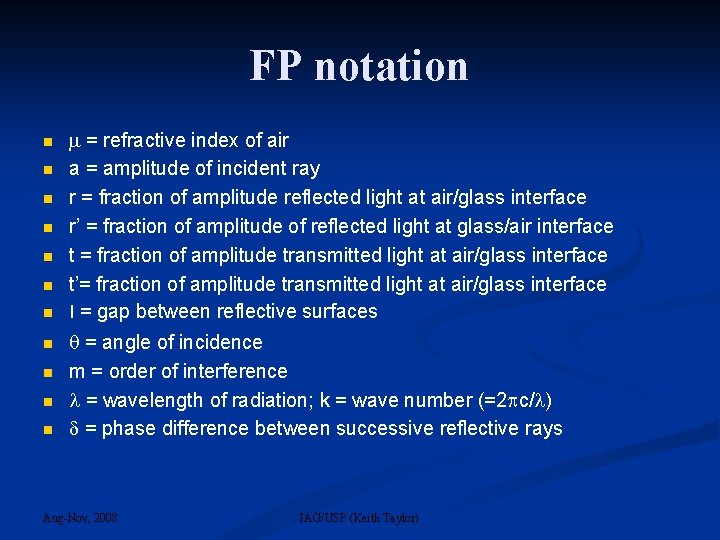FP notation = refractive index of air a = amplitude of incident ray r = fraction of amplitude reflected light at air/glass interface r’ = fraction of amplitude of reflected light at glass/air interface t = fraction of amplitude transmitted light at air/glass interface t’= fraction of amplitude transmitted light at air/glass interface l = gap between reflective surfaces = angle of incidence m = order of interference = wavelength of radiation; k = wave number (=2 c/ ) = phase difference between successive reflective rays Aug-Nov, 2008 IAG/USP (Keith Taylor)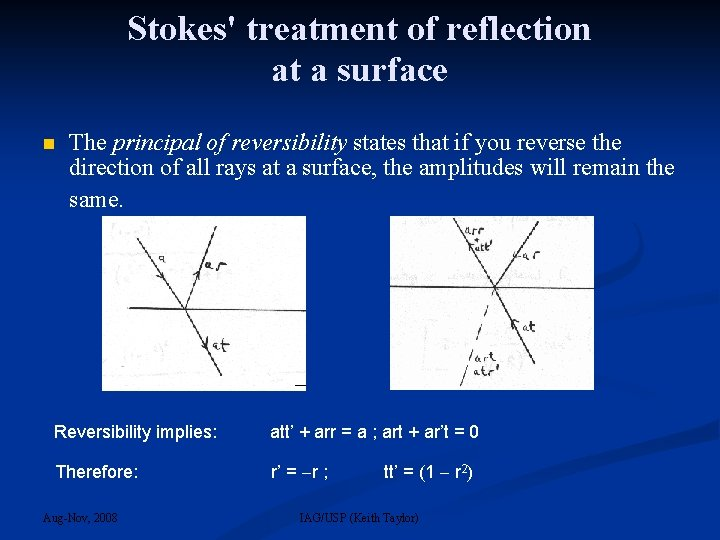Stokes' treatment of reflection at a surface The principal of reversibility states that if you reverse the direction of all rays at a surface, the amplitudes will remain the same. Reversibility implies: att’ + arr = a ; art + ar’t = 0 Therefore: r’ = r ; Aug-Nov, 2008 tt’ = (1 r 2) IAG/USP (Keith Taylor)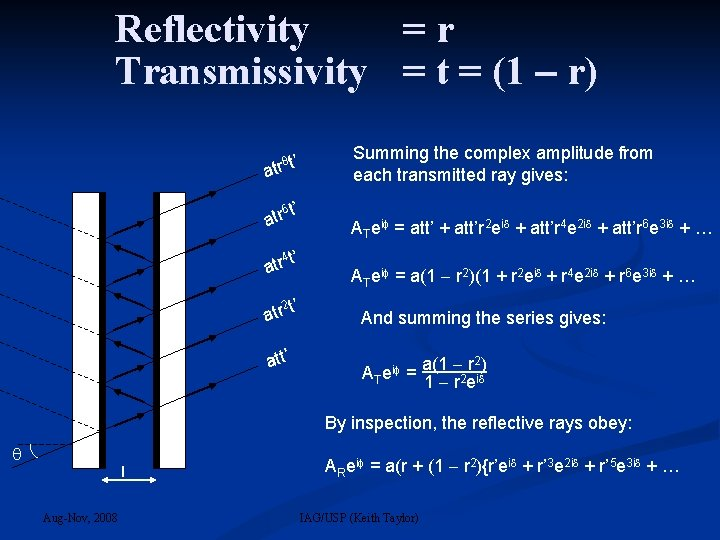Reflectivity =r Transmissivity = t = (1 r) 8 t’ atr 6 t’ atr 4 t’ atr 2 t’ atr att’ Summing the complex amplitude from each transmitted ray gives: ATei = att’ + att’r 2 ei + att’r 4 e 2 i + att’r 6 e 3 i + … ATei = a(1 r 2)(1 + r 2 ei + r 4 e 2 i + r 6 e 3 i + … And summing the series gives: 2) ATei = a(1 r 2 ei By inspection, the reflective rays obey: l Aug-Nov, 2008 ARei = a(r + (1 r 2){r’ei + r’ 3 e 2 i + r’ 5 e 3 i + … IAG/USP (Keith Taylor)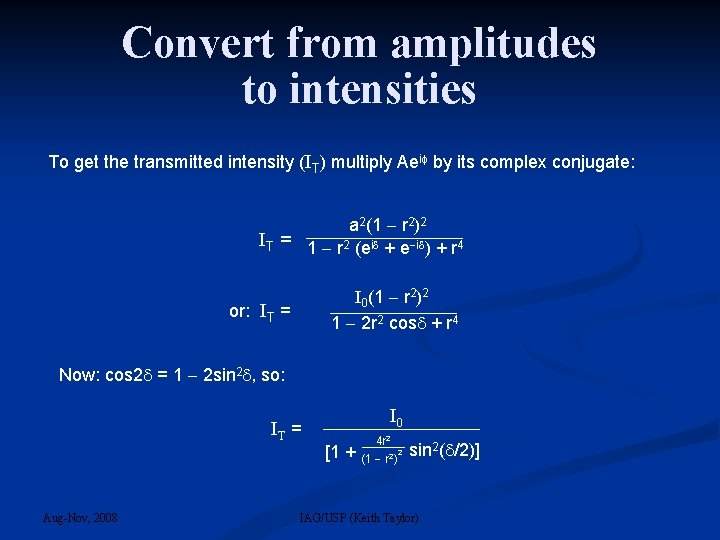Convert from amplitudes to intensities To get the transmitted intensity (IT) multiply Aei by its complex conjugate: a 2(1 r 2)2 IT = 1 r 2 (ei + e i ) + r 4 I 0(1 r 2)2 1 2 r 2 cos + r 4 or: IT = Now: cos 2 = 1 2 sin 2 , so: IT = Aug-Nov, 2008 I 0 [1 + 4 r 2 2 (1 r 2) sin 2( /2)] IAG/USP (Keith Taylor)… from which we can derive the Airy Function The phase difference is given by: = 2. 2 d. cos … and the reflected intensity R = r 2 So … IT = I 0 1 + 4 R 2 (1 R) sin 2( The FP Airy Function Aug-Nov, 2008 IAG/USP (Keith Taylor) ) 2 d. cos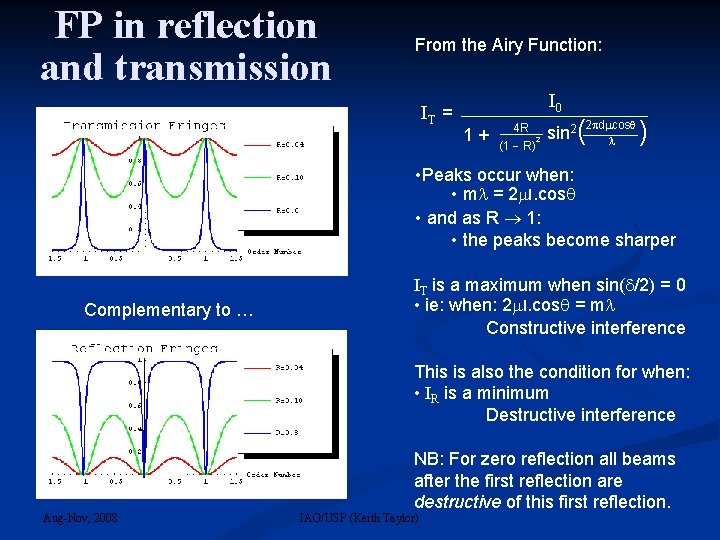FP in reflection and transmission From the Airy Function: IT = I 0 2 d cos 4 R 1 + (1 R)2 sin 2( ) • Peaks occur when: • m = 2 l. cos • and as R 1: • the peaks become sharper Complementary to … IT is a maximum when sin( /2) = 0 • ie: when: 2 l. cos = m Constructive interference This is also the condition for when: • IR is a minimum Destructive interference Aug-Nov, 2008 NB: For zero reflection all beams after the first reflection are destructive of this first reflection. IAG/USP (Keith Taylor)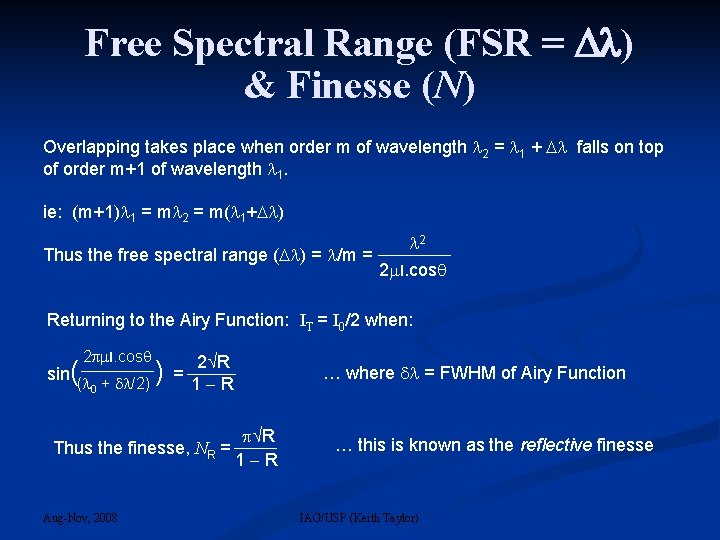Free Spectral Range (FSR = ) & Finesse (N) Overlapping takes place when order m of wavelength 2 = 1 + falls on top of order m+1 of wavelength 1. ie: (m+1) 1 = m 2 = m( 1+ ) 2 Thus the free spectral range ( ) = /m = 2 l. cos Returning to the Airy Function: IT = I 0/2 when: 2 l. cos 2 R sin(( + /2) ) = 1 R 0 R Thus the finesse, NR = 1 R Aug-Nov, 2008 … where = FWHM of Airy Function … this is known as the reflective finesse IAG/USP (Keith Taylor)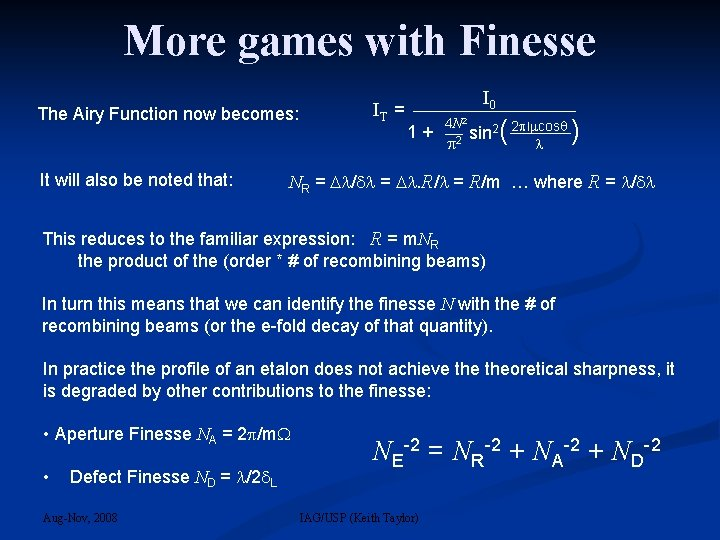More games with Finesse The Airy Function now becomes: IT = 1 + I 0 4 N 2 2 sin 2( 2 l cos ) It will also be noted that: NR = / = . R/ = R/m … where R = / This reduces to the familiar expression: R = m. NR the product of the (order * # of recombining beams) In turn this means that we can identify the finesse N with the # of recombining beams (or the e-fold decay of that quantity). In practice the profile of an etalon does not achieve theoretical sharpness, it is degraded by other contributions to the finesse: • Aperture Finesse NA = 2 /m • Defect Finesse ND = /2 L Aug-Nov, 2008 NE-2 = NR-2 + NA-2 + ND-2 IAG/USP (Keith Taylor)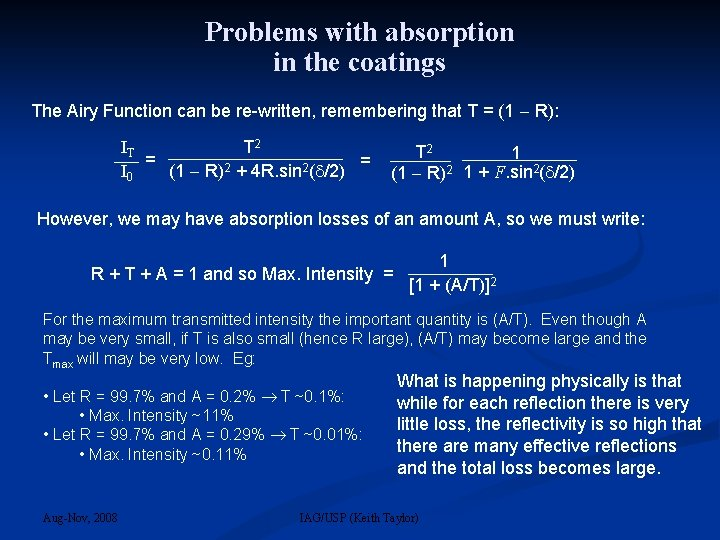Problems with absorption in the coatings The Airy Function can be re-written, remembering that T = (1 R): IT T 2 1 = = 2 2 I 0 (1 R) + 4 R. sin ( /2) (1 R)2 1 + F. sin 2( /2) However, we may have absorption losses of an amount A, so we must write: 1 R + T + A = 1 and so Max. Intensity = [1 + (A/T)]2 For the maximum transmitted intensity the important quantity is (A/T). Even though A may be very small, if T is also small (hence R large), (A/T) may become large and the Tmax will may be very low. Eg: • Let R = 99. 7% and A = 0. 2% T ~0. 1%: • Max. Intensity ~11% • Let R = 99. 7% and A = 0. 29% T ~0. 01%: • Max. Intensity ~0. 11% Aug-Nov, 2008 What is happening physically is that while for each reflection there is very little loss, the reflectivity is so high that there are many effective reflections and the total loss becomes large. IAG/USP (Keith Taylor)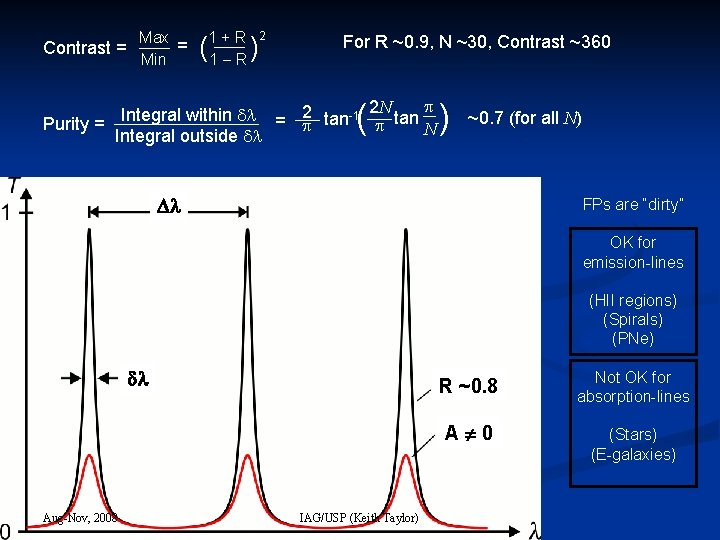Max 1 + R = Contrast = Min 1 R ( ) 2 For R ~0. 9, N ~30, Contrast ~360 2 tan-1 2 N tan Integral within = Purity = N Integral outside ( ) ~0. 7 (for all N) FPs are “dirty” OK for emission-lines (HII regions) (Spirals) (PNe) Aug-Nov, 2008 IAG/USP (Keith Taylor) R ~0. 8 Not OK for absorption-lines A 0 (Stars) (E-galaxies)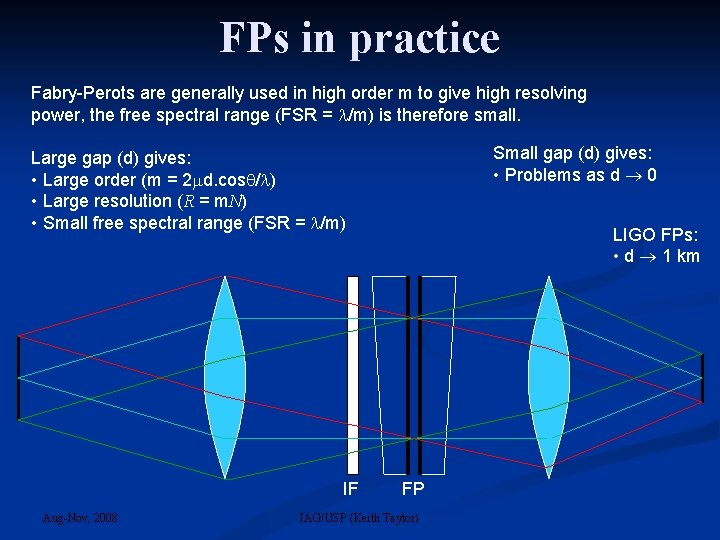FPs in practice Fabry-Perots are generally used in high order m to give high resolving power, the free spectral range (FSR = /m) is therefore small. Small gap (d) gives: • Problems as d 0 Large gap (d) gives: • Large order (m = 2 d. cos / ) • Large resolution (R = m. N) • Small free spectral range (FSR = /m) IF Aug-Nov, 2008 LIGO FPs: • d 1 km FP IAG/USP (Keith Taylor)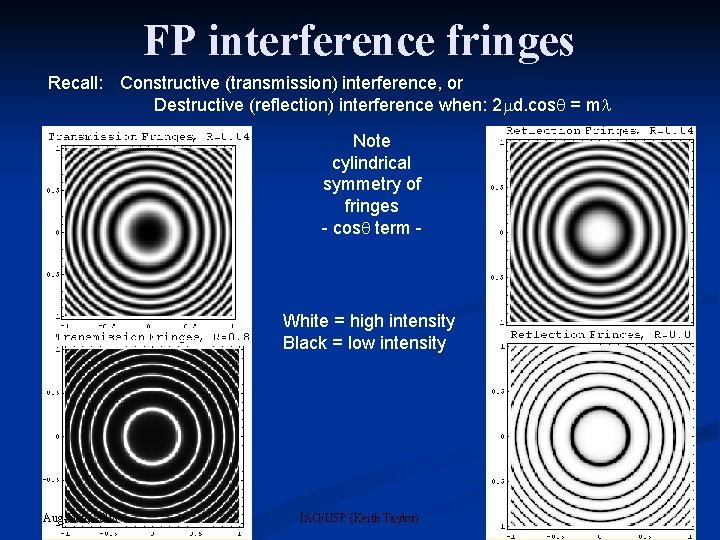FP interference fringes Recall: Constructive (transmission) interference, or Destructive (reflection) interference when: 2 d. cos = m Note cylindrical symmetry of fringes - cos term - White = high intensity Black = low intensity Aug-Nov, 2008 IAG/USP (Keith Taylor)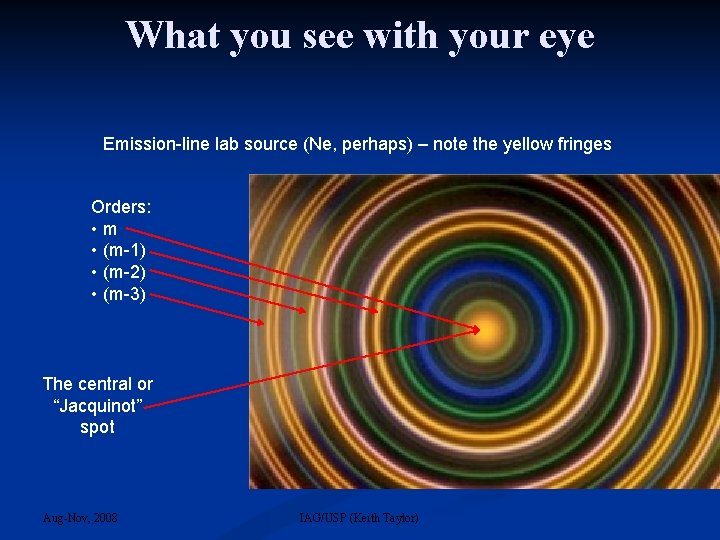What you see with your eye Emission-line lab source (Ne, perhaps) – note the yellow fringes Orders: • m • (m-1) • (m-2) • (m-3) The central or “Jacquinot” spot Aug-Nov, 2008 IAG/USP (Keith Taylor)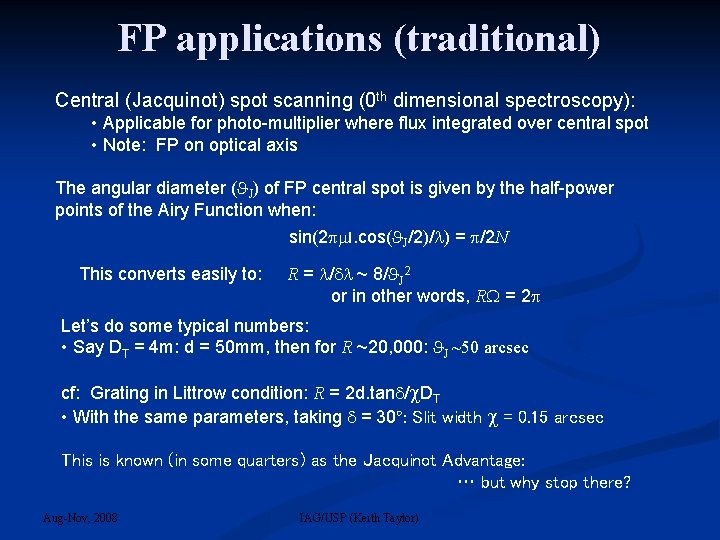FP applications (traditional) Central (Jacquinot) spot scanning (0 th dimensional spectroscopy): • Applicable for photo-multiplier where flux integrated over central spot • Note: FP on optical axis The angular diameter ( J) of FP central spot is given by the half-power points of the Airy Function when: sin(2 l. cos( J/2)/ ) = /2 N This converts easily to: R = / ~ 8/ J 2 or in other words, R = 2 Let’s do some typical numbers: • Say DT = 4 m: d = 50 mm, then for R ~20, 000: J ~50 arcsec cf: Grating in Littrow condition: R = 2 d. tan / DT • With the same parameters, taking = 30º: Slit width = 0. 15 arcsec This is known (in some quarters) as the Jacquinot Advantage: … but why stop there? Aug-Nov, 2008 IAG/USP (Keith Taylor)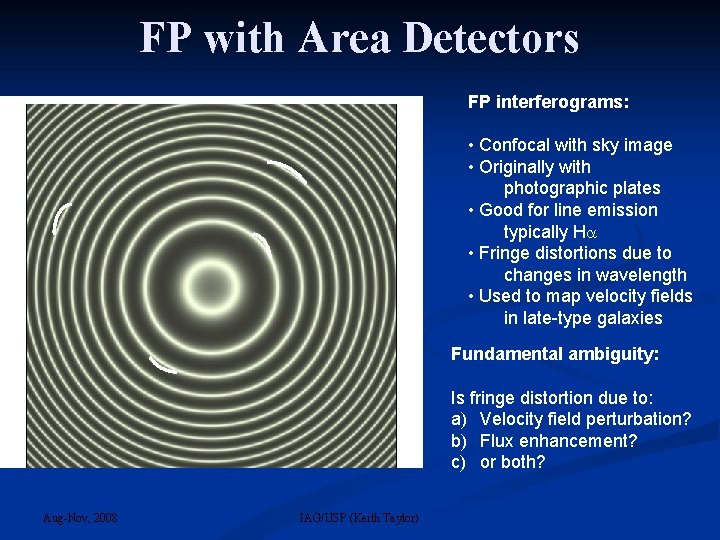FP with Area Detectors FP interferograms: • Confocal with sky image • Originally with photographic plates • Good for line emission typically H • Fringe distortions due to changes in wavelength • Used to map velocity fields in late-type galaxies Fundamental ambiguity: Is fringe distortion due to: a) Velocity field perturbation? b) Flux enhancement? c) or both? Aug-Nov, 2008 IAG/USP (Keith Taylor)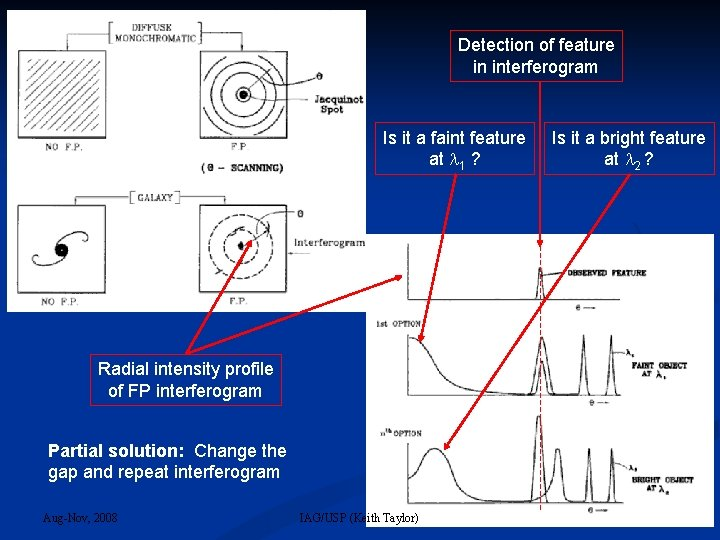Detection of feature in interferogram Is it a faint feature at 1 ? Radial intensity profile of FP interferogram Partial solution: Change the gap and repeat interferogram Aug-Nov, 2008 IAG/USP (Keith Taylor) Is it a bright feature at 2 ?Why only take 2 interferograms? Why not take 2*N? The birth of the TAURUS concept Note: A single interferogram only contains (1/N)th of in spatial information Scanning the FP across a full FSR is a natural way to: • Recover ALL of the spatial information • Resolve the fundamental ambiguity in the individual interferograms Scanning can be achieved in 3 ways: a) Changing : - by tilting the FP or by moving the image across the fringes as in photographic interferograms (to partially resolve ambiguity) b) Changing : - by changing the pressure of a gas in the FP gap as in 0 th dimensional scanning with eg: propane c) Scanning d: - by changing the physical gap between the plates very difficult since need to maintain parallelism to /2 N, at least TAURUS = QI etalons + IPCS … c 1980 Aug-Nov, 2008 IAG/USP (Keith Taylor)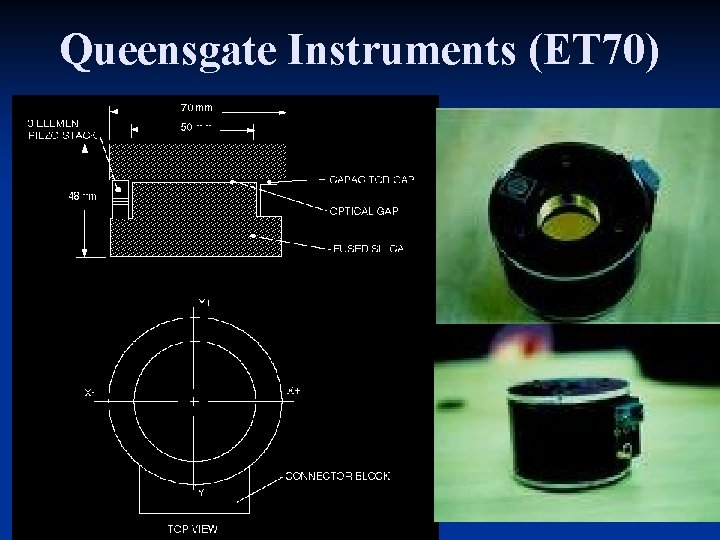Queensgate Instruments (ET 70) Aug-Nov, 2008 IAG/USP (Keith Taylor)Queensgate Instruments (Capacitance Micrometry) FP etalons Piezo transducers (3) CS 100 controller Construction • Super-flat /200 base • Centre piece (optical contact) • Top plate Capacitors • X-bridge • Y-bridge • Z-bridge (+ stonehenge) Aug-Nov, 2008 IAG/USP (Keith Taylor) Scanning d over 1*FSR m (m-1) ; d d + ( /2)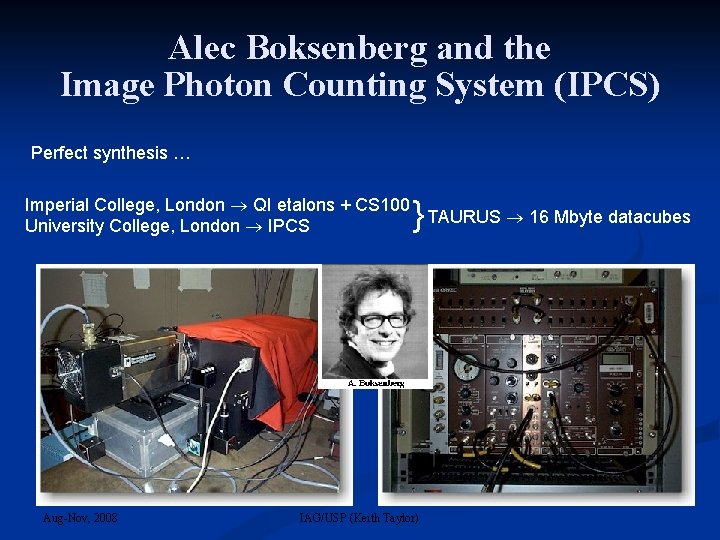Alec Boksenberg and the Image Photon Counting System (IPCS) Perfect synthesis … } Imperial College, London QI etalons + CS 100 TAURUS 16 Mbyte datacubes University College, London IPCS Aug-Nov, 2008 IAG/USP (Keith Taylor)How big a field? From before, Jacquinot central spot given by: R = 2 or: R ~ 8 2/ 2 ~ 8 2(d/DT)2/ J 2, where J is the angle on the sky. However, given an array detector, we can work off-axis: So, how far? Answer: Until the rings get narrower than ~2 pixels (the seeing disk) Now, from the Airy Function we obtain: d /d = -1/ 0. sin( ) Aug-Nov, 2008 The full TAURUS field, F is then: F = 2 2(d/DT)2/R F/ J ~ 20, typically IAG/USP (Keith Taylor) or ~400 inTAURUS Datacube Recalling the phase delay equn: m = 2 l. cos For small values of : goes as [1 – ( 2/2)] where tan-1[(y-y 0]/[x-x 0]) and (x 0, y 0) = centre of FP fringes Þ (x 1, y 1) is shifted in z-dirn w. r. t. (x 0, y 0) and this -shift is thus ~parabolic in It is also periodic in “m”. We thus refer to this shift as a “Phase-correction” So the surface of constant is a “Nested Parabola” Cut through a “Nested Parabola and you get a set of rings and these rings are the FP fringes. Aug-Nov, 2008 IAG/USP (Keith Taylor)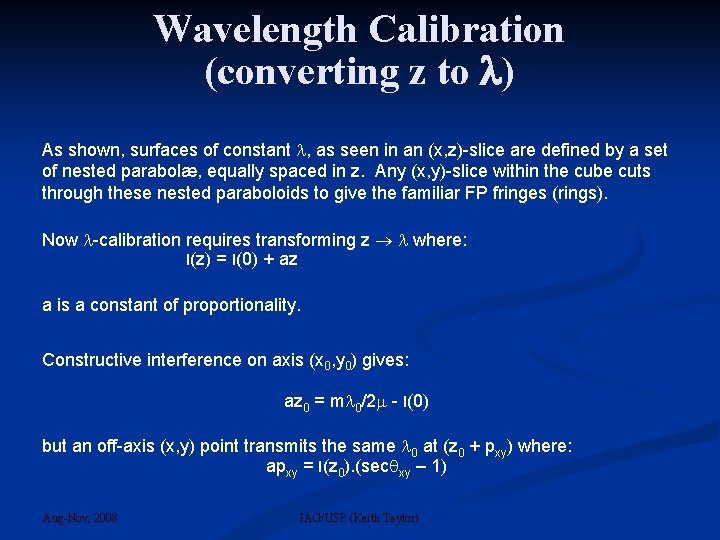Wavelength Calibration (converting z to ) As shown, surfaces of constant , as seen in an (x, z)-slice are defined by a set of nested parabolæ, equally spaced in z. Any (x, y)-slice within the cube cuts through these nested paraboloids to give the familiar FP fringes (rings). Now -calibration requires transforming z where: l(z) = l(0) + az a is a constant of proportionality. Constructive interference on axis (x 0, y 0) gives: az 0 = m 0/2 - l(0) but an off-axis (x, y) point transmits the same 0 at (z 0 + pxy) where: apxy = l(z 0). (sec xy – 1) Aug-Nov, 2008 IAG/USP (Keith Taylor)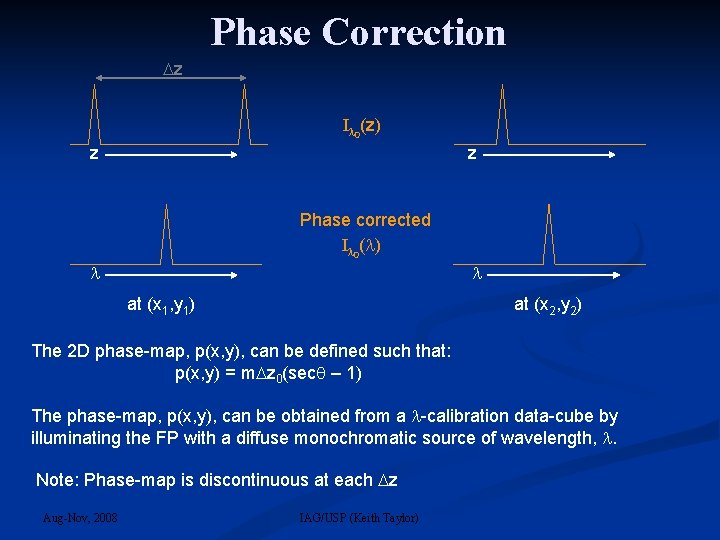Phase Correction z I 0(z) z z Phase corrected I 0( ) at (x 1, y 1) at (x 2, y 2) The 2 D phase-map, p(x, y), can be defined such that: p(x, y) = m z 0(sec – 1) The phase-map, p(x, y), can be obtained from a -calibration data-cube by illuminating the FP with a diffuse monochromatic source of wavelength, . Note: Phase-map is discontinuous at each z Aug-Nov, 2008 IAG/USP (Keith Taylor)The Phase-Map The phase-map is so called since it can be used to transform the raw TAURUS cube, with its strange multi-paraboloidal iso-wavelength contours into a welltempered data cube where all (x, y)-slices are now at constant wavelengths. The process is called phase-correction since it represents a periodic function of period, z. ie: If the z-value (z’) of a phase-shifted pixel exceeds the z-dimensions of the data-cube, then the spectra is simply folded back by one FSR to (z’ - z). It will be noted that the phase-map (as defined previously) is independent of and hence in principle any calibration wavelength, cal, can be used to phasecorrect a observation data-cube at an arbitary obs, remembering that: 2 & z = /2 But also, the phase-map can be expressed in -space as: xy = 0(1 - cos ) and hence is also independent of gap, l, and thus applicable to all FPs at all . Aug-Nov, 2008 IAG/USP (Keith Taylor)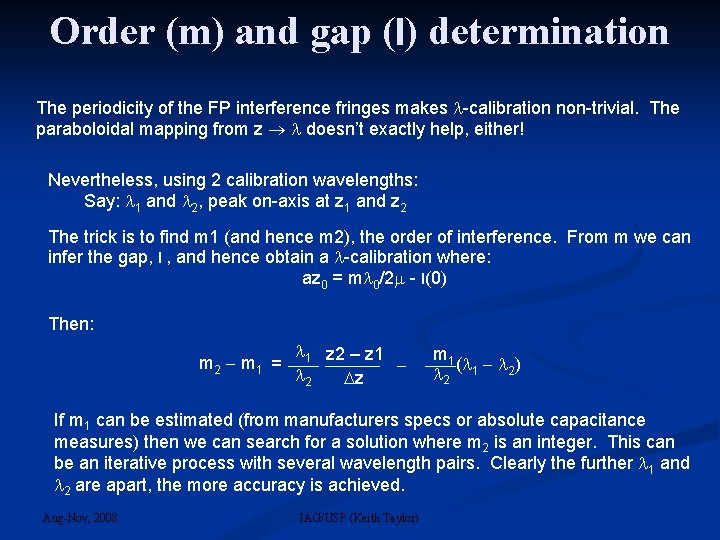Order (m) and gap (l) determination The periodicity of the FP interference fringes makes -calibration non-trivial. The paraboloidal mapping from z doesn’t exactly help, either! Nevertheless, using 2 calibration wavelengths: Say: 1 and 2, peak on-axis at z 1 and z 2 The trick is to find m 1 (and hence m 2), the order of interference. From m we can infer the gap, l , and hence obtain a -calibration where: az 0 = m 0/2 - l(0) Then: m 2 m 1 = 1 z 2 – z 1 2 z m 1 ( 2) 2 1 If m 1 can be estimated (from manufacturers specs or absolute capacitance measures) then we can search for a solution where m 2 is an integer. This can be an iterative process with several wavelength pairs. Clearly the further 1 and 2 are apart, the more accuracy is achieved. Aug-Nov, 2008 IAG/USP (Keith Taylor)Wavelength Calibration If m 1 can be estimated (from manufacturers specs or absolute capacitance measures) then we can search for a solution where m 2 is an integer. This can be an iterative process with several wavelength pairs. Clearly the further 1 and 2 are apart, the more accuracy is achieved. Once the interference order, m 1, for a known wavelength, 1, has been identified then wavelength calibration is given by: = 0 Aug-Nov, 2008 (z z ) + 1 { } m z 0 0 0 IAG/USP (Keith Taylor)FPP in collimated beam • Interference fringes formed at infinity: • Sky and FP fringes are con-focal • Detector sees FP fringes superimposed on sky image IF Aug-Nov, 2008 FP IAG/USP (Keith Taylor)FPI in image plane • Interference fringes formed at infinity: • Sky and FP fringes are not con-focal • Detector sees FP plates superimposed on sky image • ie: No FP fringes seen on detector • FP is not perfectly centred on image plane (out of focus) to avoid detector seeing dust particles on plates. FP IF Aug-Nov, 2008 IAG/USP (Keith Taylor)FP (or Interference Filter) in image plane The FP still acts as a periodic monochromator but the angles into the FP (or IF) must not exceed the Jacquinot criteria, which states that: 2 < 8 2/R (or R = 2 ) At f/8: = 2. tan-1(1/16) ~7. 2º, so R < 500 At f/16: = 2. tan-1(1/32) ~3. 6º, so R < 2, 000 Note: the Fo. V is determined not by the width of the fringes but by the diameter of the FP. Also, an IF is simply a solid FP ( ~2. 1) with very narrow gap. Aug-Nov, 2008 IAG/USP (Keith Taylor)Aug-Nov, 2008 IAG/USP (Keith Taylor)FB observations of NGC 7793 on the 3. 60 m. Top left: DSS Blue Band image. Top right: Spitzer infrared array camera (IRAC) 3. 6 μm image. Middle left: Hα monochromatic image. Middle right: Hα velocity field. Bottom: position-velocity (PV) diagram. Aug-Nov, 2008 IAG/USP (Keith Taylor)FB observations of NGC 7793 on the 36 cm. Top left: DSS Blue Band image. Top right: Spitzer IRAC 3. 6 μm image. Middle left: Hα monochromatic image. Middle right: Hα velocity field. Bottom: PV diagram. Aug-Nov, 2008 IAG/USP (Keith Taylor)ADHOC screen shot (Henri) Aug-Nov, 2008 IAG/USP (Keith Taylor)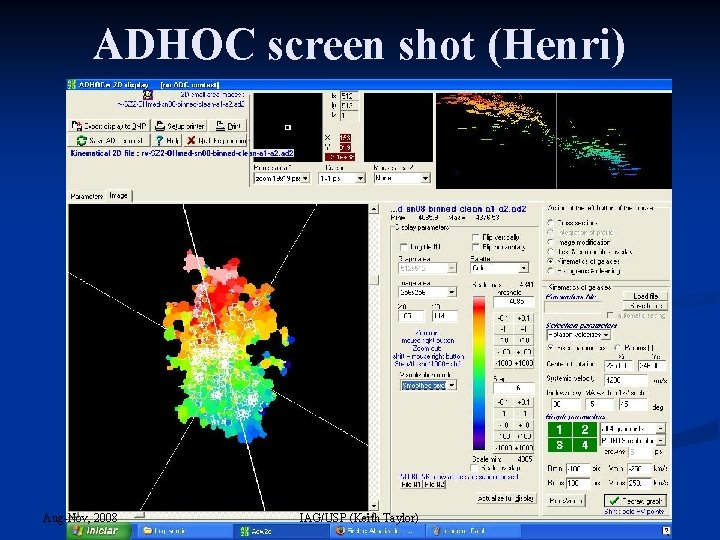ADHOC screen shot (Henri) Aug-Nov, 2008 IAG/USP (Keith Taylor)